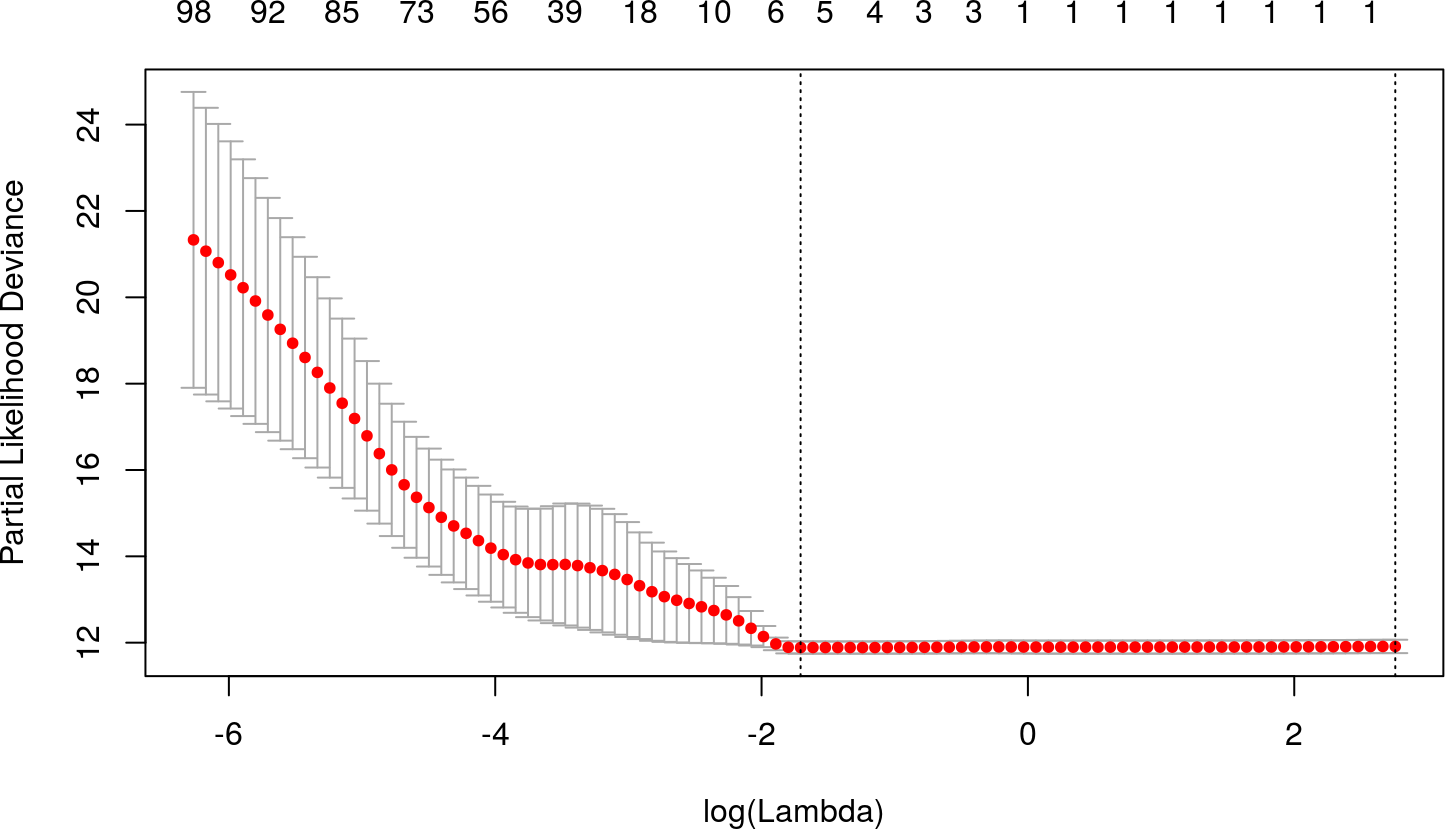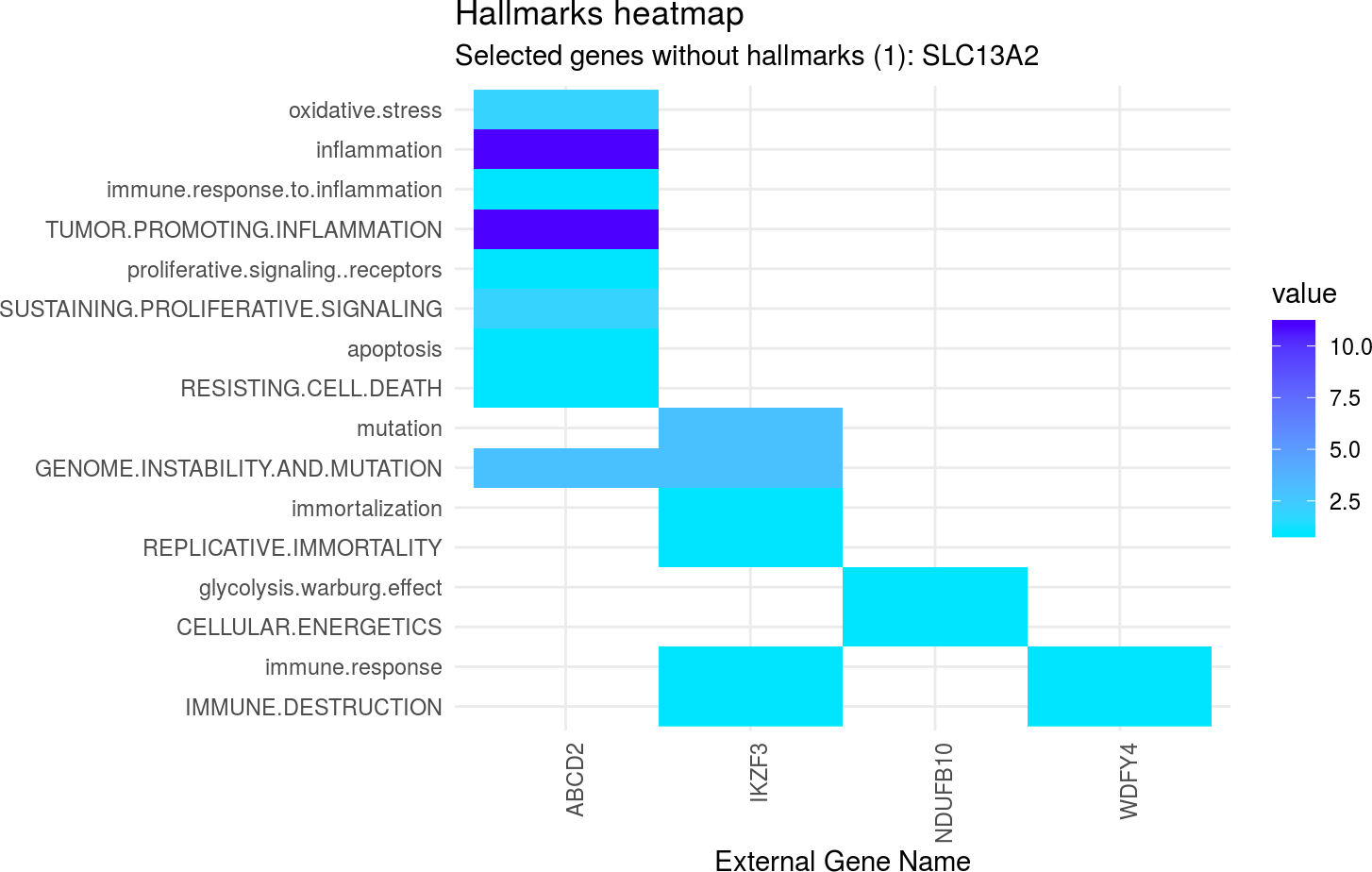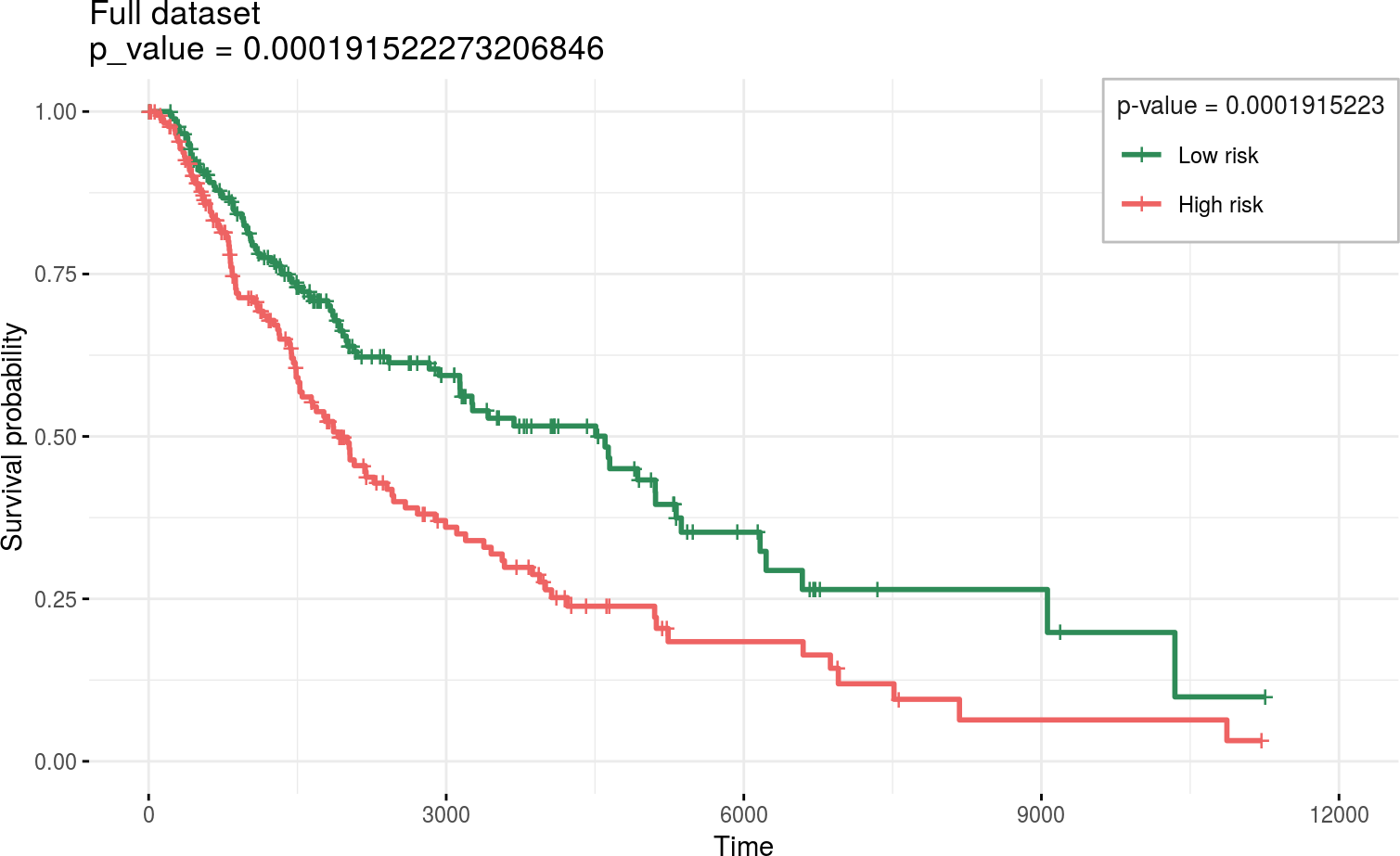# Contents

## 0.1 Instalation

if (!require('BiocManager'))
install.packages('BiocManager')
BiocManager::install('glmSparseNet')

# 1 Required Packages

library(dplyr)
library(ggplot2)
library(survival)
library(loose.rock)
library(futile.logger)
library(TCGAutils)
#
library(glmSparseNet)
#
# Some general options for futile.logger the debugging package
.Last.value <- flog.layout(layout.format('[~l] ~m'))
.Last.value <- loose.rock::show.message(FALSE)
# Setting ggplot2 default theme as minimal
theme_set(ggplot2::theme_minimal())

# 2 Load data

The data is loaded from an online curated dataset downloaded from TCGA using curatedTCGAData bioconductor package and processed.

To accelerate the process we use a very reduced dataset down to 107 variables only (genes), which is stored as a data object in this package. However, the procedure to obtain the data manually is described in the following chunk.

skcm <- curatedTCGAData(diseaseCode = 'SKCM', assays = 'RNASeq2GeneNorm', FALSE)

Build the survival data from the clinical columns.

• Merge survival times for patients, which have different columns in case they are alive or dead.
• Build two matrix objects that fit the data xdata and ydata
skcm.metastatic <- TCGAutils::splitAssays(skcm, '06')
## Selecting 'Metastatic' samples
xdata.raw <- t(assay(skcm.metastatic[]))

# Get survival information
ydata.raw <- colData(skcm.metastatic) %>% as.data.frame %>%
# Find max time between all days (ignoring missings)
rowwise %>%
mutate(time = max(days_to_last_followup, days_to_death, na.rm = TRUE)) %>%
# Keep only survival variables and codes
select(patientID, status = vital_status, time) %>%
# Discard individuals with survival time less or equal to 0
filter(!is.na(time) & time > 0) %>% as.data.frame

# Get survival information
ydata.raw <- colData(skcm) %>% as.data.frame %>%
# Find max time between all days (ignoring missings)
rowwise %>%
mutate(time = max(days_to_last_followup, days_to_death, na.rm = TRUE)) %>%
# Keep only survival variables and codes
select(patientID, status = vital_status, time) %>%
# Discard individuals with survival time less or equal to 0
filter(!is.na(time) & time > 0) %>% as.data.frame

# Set index as the patientID
rownames(ydata.raw) <- ydata.raw$patientID # keep only features that have standard deviation > 0 xdata.raw <- xdata.raw[TCGAbarcode(rownames(xdata.raw)) %in% rownames(ydata.raw),] xdata.raw <- xdata.raw %>% { (apply(., 2, sd) != 0) } %>% { xdata.raw[, .] } %>% scale # Order ydata the same as assay ydata.raw <- ydata.raw[TCGAbarcode(rownames(xdata.raw)), ] set.seed(params$seed)
small.subset <- c('FOXL2', 'KLHL5', 'PCYT2', 'SLC6A10P', 'STRAP', 'TMEM33',
'WT1-AS', sample(colnames(xdata.raw), 100))

xdata <- xdata.raw[, small.subset[small.subset %in% colnames(xdata.raw)]]
ydata <- ydata.raw %>% select(time, status)

# 3 Fit models

Fit model model penalizing by the hubs using the cross-validation function by cv.glmHub.

fitted <- cv.glmHub(xdata,
Surv(ydata$time, ydata$status),
family  = 'cox',
foldid  = balanced.cv.folds(!!ydata$status)$output,
network = 'correlation',
network.options = networkOptions(min.degree = .2,
cutoff = .6))

# 4 Results of Cross Validation

Shows the results of 100 different parameters used to find the optimal value in 10-fold cross-validation. The two vertical dotted lines represent the best model and a model with less variables selected (genes), but within a standard error distance from the best.

plot(fitted)## 4.1 Coefficients of selected model from Cross-Validation

Taking the best model described by lambda.min

coefs.v <- coef(fitted, s = 'lambda.min')[,1] %>% { .[. != 0]}
coefs.v %>% {
data.frame(ensembl.id  = names(.),
gene.name   = geneNames(names(.))$external_gene_name, coefficient = ., stringsAsFactors = FALSE) } %>% arrange(gene.name) %>% knitr::kable() ensembl.id gene.name coefficient IKZF3 ABCD2 0.1106294 ABCD2 IKZF3 -0.2870459 SLC13A2 NDUFB10 0.0345453 WDFY4 SLC13A2 -0.0517820 NDUFB10 WDFY4 0.0881267 ## 4.2 Hallmarks of Cancer geneNames(names(coefs.v)) %>% { hallmarks(.$external_gene_name)$heatmap }## 4.3 Survival curves and Log rank test separate2GroupsCox(as.vector(coefs.v), xdata[, names(coefs.v)], ydata, plot.title = 'Full dataset', legend.outside = FALSE) ##$pvalue
##  0.0001915223
##
## $plot## ##$km
## Call: survfit(formula = survival::Surv(time, status) ~ group, data = prognostic.index.df)
##
##             n events median 0.95LCL 0.95UCL
## Low risk  180     83   4601    3139    5318
## High risk 179    110   1910    1525    2470